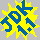SpiroAnimexample

SpiroAnim is a Java 1.1 Component that displays an animation of a SpiroGraph. It's very easy to use. Just construct a SpiroAnim object and add it to a Container. The animation will start automatically. The animation thread has a low priority so it will not disturb important threads.

The component is ideal to be displayed in an applet while the user is waiting for some data to be loaded.

What is a Spirograph?

A Spirograph is formed by rolling a circle inside or outside of another circle. The pen is placed at any point on the rolling circle. If the radius of fixed circle is R, the radius of moving circle is r, and the offset of the pen point in the moving circle is O, then the equation of the resulting curve is defined by:

x = (R+r)*cos(t) - (r+O)*cos(((R+r)/r)*t)
y = (R+r)*sin(t) - (r+O)*sin(((R+r)/r)*t)

SpiroAnim draws a line between points defined by t and t+1. Then between the points defined by t+1 and t+2 and so on.

How to use this component in your applications?

It is very easy to use the SpiroAnim. Just add the component to a panel and you're off. This can be done like this:
SpiroAnim sa = new SpiroAnim();